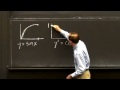### Total 18 Videos found in Category "Highlights of Calculus"

Title
 1Big Picture of Calculus 2Big Picture: Derivatives 3Big Picture: Integrals 4Chains f(g(x)) and the Chain Rule | MIT Highlights of Calculus 5Derivative of sin x and cos x | MIT Highlights of Calculus 6Derivatives of ln y and sin ^-1 (y) | MIT Highlights of Calculus 7Differential Equations of Growth | MIT Highlights of Calculus 8Differential Equations of Motion | MIT Highlights of Calculus 9Euler Great Formula | MIT Highlights of Calculus 10Gil Strang Introduction to Calculus for Highlights for High School 11Growth Rates and Log Graphs 12Inverse Funtions f ^-1 (y) and the Logarithm x = ln y | MIT Highlights of Calculus 13Limits and Continuous Functions | MIT Highlights of Calculus 14Linear Approximation/Netwon Method | MIT Highlights of Calculus 15Max and Min and Second Derivative 16Product Rule and Quotient Rule | MIT Highlights of Calculus 17Six Functions, Six Rules, and Six Theorems | MIT Highlights of Calculus 18The Exponential Function

Say and share some thing about these videos...